# Visualizing 3 D In 2 D Solution of TS & AP Board Class 8 Mathematics

###### Exercise 13.1

Question 1.

Draw the following 3-D figures on isometric dot sheet.

(i)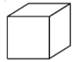(ii)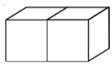(iii)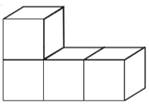(iv)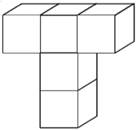(i)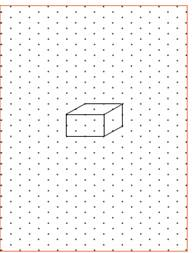(ii)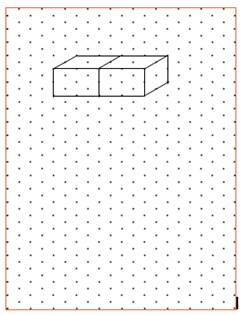(iii)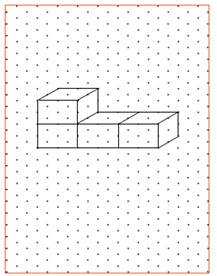(iv)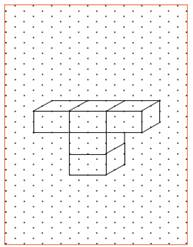Question 2.

Draw a cuboid on the isometric dot sheet with the measurements 5 units × 3 units × 2 units.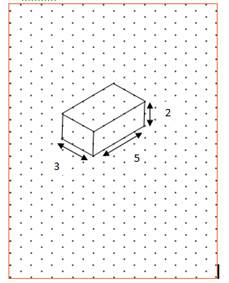Question 3.

Find the number of unit cubes in the following 3-D figures.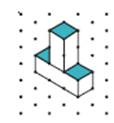A unit cube is drawn by joining 2 adjacent dots in the isometric sheet.

Here in the vertical bar, there are 2 unit cubes

In the horizontal bar, there are 3 unit cubes

∴ Total no of unit cubes in given figure is: 5

Question 4.

Find the number of unit cubes in the following 3-D figures.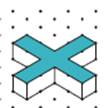Here, in each bar there are 2 unit cubes

∴ 2× 4(bars) = 8 unit cubes

And also there is a unit cube in center

∴ Total no. of unit cubes in given figure are: 8 + 1 = 9

Question 5.

Find the number of unit cubes in the following 3-D figures.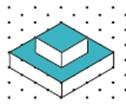Here, there are 4 unit cubes in the top layer

In bottom layer, there are 4 + 12 = 16 unit cubes

∴ Total no. of unit cubes in the given figure are:20

Question 6.

Find the number of unit cubes in the following 3-D figures.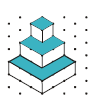Here, there is 1 cube at the top

In the middle layer, there are 4 cubes

In the bottom layer, there are 9 cubes

∴ Total no. of cubes = 1 + 4 + 9 = 14 unit cubes

Question 7.

Consider the distance between two consecutive dots to be 1 cm and draw the front view, side view and top view of the following 3-D figures.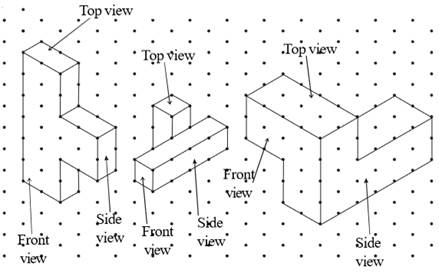(a)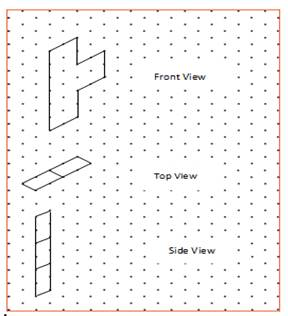(b)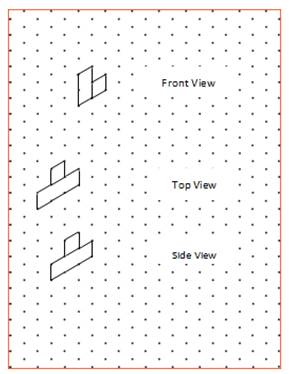(C)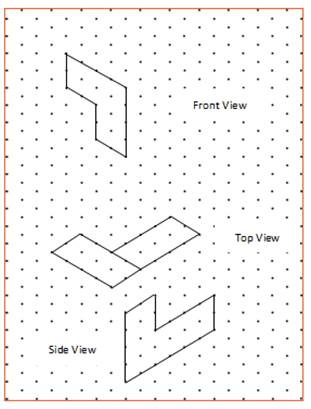Question 8.

Find the areas of the shaded regions of the 3-D figures given in question number 3.

(i)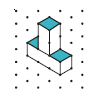In the figure we can clearly see that there are 3 shaded squares with 1 sq. unit area

∴ total shaded area = 3×1 sq. units = 3 sq. units

(ii)Here, we can clearly see that all upper faces of the cubes in the figure are shaded and there are 9 unit cubes in the figure.

∴ area of the shaded region = 9×1 sq. unit = 9 sq units

(iii)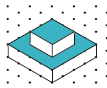Here, we can see that whole upper face of top layer unit cubes is shaded, that is 4 unit cubes and in bottom layer, we can see that upper face of 12 unit cubes are shaded.

∴ Total shaded area = (4 + 12)× 1 sq. unit = 16 sq. units

(iv)Here, we can see that upper face of the unit cube in the top layer is shaded and in middle layer 4 unit cubes are shaded and in the bottom layer, 5 unit cubes are shaded.

∴ total shaded area = (1 + 3 + 5)× 1 sq. unit = 8 sq. units

###### Exercise 13.2

Question 1.

Count the number of faces , vertices , and edges of given polyhedra and verify Euler’s formula.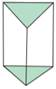Here,

No. of Faces (F):5

No. of Vertices (V):6

No. of Edges (E):9

Euler’s formula: F + V = E + 2

⇒ 5 + 6 = 9 + 2

⇒ 11 = 11

∴ Euler’s formula satisfies for the shape.

Question 2.

Count the number of faces , vertices , and edges of given polyhedra and verify Euler’s formula.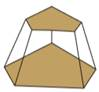Here,

No. of Faces (F):7

No. of Vertices (V):10

No. of Edges (E):15

Euler’s formula: F + V = E + 2

⇒ 7 + 10 = 15 + 2

⇒ 17 = 17

∴ Euler’s formula satisfies for the shape.

Question 3.

Count the number of faces , vertices , and edges of given polyhedra and verify Euler’s formula.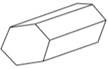Here,

No. of Faces (F):8

No. of Vertices (V):12

No. of Edges (E):18

Euler’s formula: F + V = E + 2

⇒ 8 + 12 = 18 + 2

⇒ 20 = 20

∴ Euler’s formula satisfies for the shape.

Question 4.

Count the number of faces , vertices , and edges of given polyhedra and verify Euler’s formula.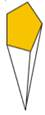Here,

No. of Faces (F):6

No. of Vertices (V):6

No. of Edges (E):10

Euler’s formula: F + V = E + 2

⇒ 6 + 6 = 10 + 2

⇒ 12 = 12

∴ Euler’s formula satisfies for the shape.

Question 5.

Count the number of faces , vertices , and edges of given polyhedra and verify Euler’s formula.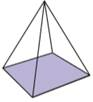Here,

No. of Faces (F):5

No. of Vertices (V):5

No. of Edges (E):8

Euler’s formula: F + V = E + 2

⇒ 5 + 5 = 8 + 2

⇒ 10 = 10

∴ Euler’s formula satisfies for the shape.

Question 6.

Count the number of faces , vertices , and edges of given polyhedra and verify Euler’s formula.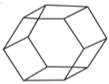Here,

No. of Faces (F):8

No. of Vertices (V):12

No. of Edges (E):18

Euler’s formula: F + V = E + 2

⇒ 8 + 12 = 18 + 2

⇒ 20 = 20

∴ Euler’s formula satisfies for the shape.

Question 7.

Count the number of faces , vertices , and edges of given polyhedra and verify Euler’s formula.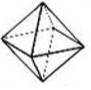Here,

No. of Faces (F):8

No. of Vertices (V):6

No. of Edges (E):12

Euler’s formula: F + V = E + 2

⇒ 8 + 6 = 12 + 2

⇒ 14 = 14

∴ Euler’s formula satisfies for the shape.

Question 8.

Count the number of faces , vertices , and edges of given polyhedra and verify Euler’s formula.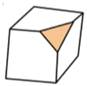Here,

No. of Faces (F):7

No. of Vertices (V):10

No. of Edges (E):15

Euler’s formula: F + V = E + 2

⇒ 7 + 10 = 15 + 2

⇒ 17 = 17

∴ Euler’s formula satisfies for the shape.

Question 9.

Is a square prism and cube are same? explain.

No, Every Square prism is not a Cube (∵ All faces in a square prism need not be identical), but conversely all cubes are Square prisms.

Question 10.

Can a polyhedra have 3 triangular faces only? explain.

No, a polyhedra with 3 triangular faces does not exist,

∵For a polyhedra with 3 triangular faces, there exist another triangular face.

Question 11.

Can a polyhedra have 4 triangular faces only? explain.

Yes, a polyhedra with exactly 4 triangular faces can exist,

A polyhedra with 4 triangular faces is triangular Pyramid.

Question 12.

Complete the table by using Euler’s formula.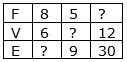(i) Given: Faces = 8

Vertices = 6

Edges = ?

According to Euler’s formula, we know that : F + V = E + 2

∴ 8 + 6 = E + 2

⇒ 14 = E + 2

⇒ E = 14—2 = 12

∴ No. of Edges = 12

(ii) Given: Faces = 5

Vertices = ?

Edges = 9

According to Euler’s formula, we know that : F + V = E + 2

∴ 5 + V = 9 + 2

⇒ 5 + V = 11

⇒ V = 11—5 = 6

∴ No. of Vertices = 6

(iii) Given: Faces = ?

Vertices = 12

Edges = 30

According to Euler’s formula, we know that : F + V = E + 2

∴ F + 12 = 30 + 2

⇒ F + 12 = 32

⇒ E = 32—12 = 20

∴ No. of faces = 20

Question 13.

Can a polyhedra have 10 faces, 20 edges and 15 vertices?

Given: No. of face(F):10

No. of edges(E):20

No. of vertices(V):15

According to Euler formula, we know that

F + V = E + 2

That is, here

10 + 15 = 20 + 2

⇒ 25 = 22

This is not true.(That is, 25≠22 )

∴ A polyhedra with 10 faces, 20 edges and 15 vertices is not possible.

Question 14.

Complete the following table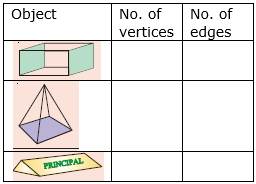1) A cuboid has 8 vertices and 12 edges.

2) A square pyramid has 5 vertices and 8 edges.

3) A triangular prism has 6 vertices and 9 edges.

∴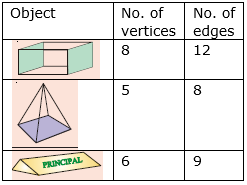Question 15.

Name the 3-D objects or shapes that can be formed from the following nets.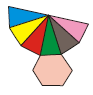No. of faces in the net: 7 (1 hexagon + 6 triangles)

No. of vertices in the net: 7

No. of edges in the net: 12

∴The possible shape we get from the net is hexagonal pyramid.

Question 16.

Name the 3-D objects or shapes that can be formed from the following nets.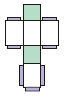No. of faces in the net: 6 (2 squares + 4 rectangles)

No. of vertices in the net: 8

No. of edges in the net: 12

∴The possible shape we get from the net is Cuboid.

Question 17.

Name the 3-D objects or shapes that can be formed from the following nets.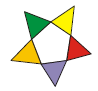No. of faces in the net:6(1 pentagon + 5 triangles)

No. of vertices in the net: 6

No. of edges in the net: 10

∴The possible shape we get from the net is pentagonal pyramid.

Question 18.

Name the 3-D objects or shapes that can be formed from the following nets.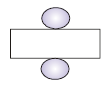No. of faces in the net: 3 (2 circles + 1 rectangle)

No. of vertices in the net: 0

No. of edges in the net: 2

∴The possible shape we get from the net is Cylinder.

Question 19.

Name the 3-D objects or shapes that can be formed from the following nets.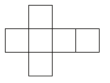No. of faces in the net: 6(6 squares)

No. of vertices in the net: 8

No. of edges in the net: 12

∴The possible shape we get from the net Cube.

Question 20.

Name the 3-D objects or shapes that can be formed from the following nets.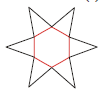No. of faces in the net:6(1 hexagon + 6 triangles)

No. of vertices in the net: 7

No. of edges in the net: 12

∴The possible shape we get from the net is hexagonal pyramid.

Question 21.

Name the 3-D objects or shapes that can be formed from the following nets.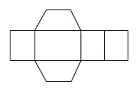No. of faces in the net:6(2 trapezium + 4 rectangles)

No. of vertices in the net: 8

No. of edges in the net: 12

∴The possible shape we get from the net is trapezoid.

Question 22.

Draw the following diagram on the check ruled book and find out which of the following diagrams makes cube?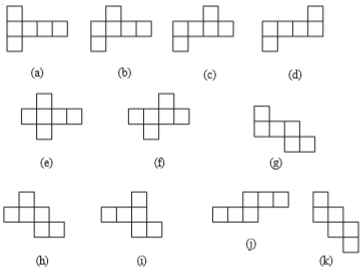(a)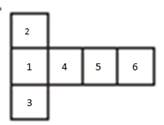i. Fold Square-2, 90° downwards

ii. Fold Square-3, 90° downwards

iii. Fold Square-4, 90° downwards

iv. Now, fold Square-5, 90° left upward

v. Now, fold Square-6, 90° upward

Hence, by the above procedure, we can form a cube with the given net.

(b)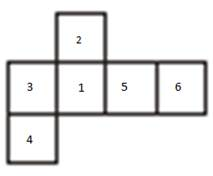i. Fold Square-2, 90° downwards

ii. Fold Square-3, 90° downwards

iii. Fold Square-4, 90° right

iv. Now, fold Square-5, 90° downward

v. Now, fold Square-6, 90° left upward

Hence, by the above procedure, we can form a cube with the given net.

(c)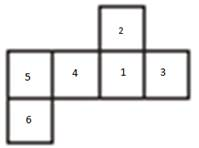i. Fold Square-2, 90° downwards

ii. Fold Square-3, 90° downwards

iii. Fold Square-4, 90° downwards

iv. Now, fold Square-5, 90° right upward

v. Now, fold Square-6, 90° right

Hence, by the above procedure we can form a cube with the given net.

(d)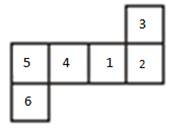i. Fold Square-2, 90° downwards

ii. Fold Square-3, 90° left

iii. Fold Square-4, 90° downwards

iv. Now, fold Square-5, 90° right upward

v. Now, fold Square-6, 90° right

Hence, by the above procedure we can form a cube with the given net.

(e)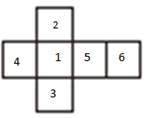i. Fold Square-2, 90° downwards

ii. Fold Square-3, 90° downwards

iii. Fold Square-4, 90° downwards

iv. Fold Square-5, 90° downward

v. Now, fold Square-6, 90° left upward

Hence, by the above procedure we can form a cube with the given net.

(f)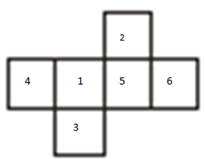i. Fold Square-3, 90° downwards

ii. Fold Square-4, 90° downwards

iii. Fold Square-5, 90° downwards

iv. Now, fold Square-2 90° left

v. Now, fold Square-6 90° left upward

Hence, by the above procedure we can form a cube with the given net.

(g)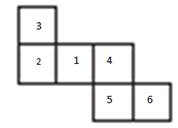i. Fold Square-2, 90° downwards

ii. Fold Square-3, 90° right

iii. Fold Square-4, 90° downwards

iv. Now, fold Square-5 90° left

v. Now, fold Square-6 90° straight upward

Hence, by the above procedure we can form a cube with the given net.

(h)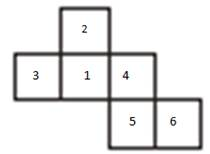i. Fold Square-2, 90° downwards

ii. Fold Square-3, 90° downwards

iii. Fold Square-4, 90° downwards

iv. Now, fold Square-5 90° left

v. Now, fold Square-6 90° straight upward

Hence, by the above procedure we can form a cube with the given net.

(i)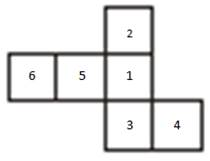i. Fold Square-2, 90° downwards

ii. Fold Square-3, 90° downwards

iii. Fold Square-4, 90° left of square-3

iv. Now, fold Square-5 90° downwards

v. Now, fold Square-6 90° right upward

Hence, by the above procedure we can form a cube with the given net.

(j)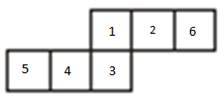i. Fold Square-2, 90° downwards

ii. Fold Square-3, 90° downwards

iii. Fold Square-4, 90° right to square-3

iv. Now, fold Square-5 90° right to square-4

v. Now, fold Square-6 90° left upward

Hence, by the above procedure we can form a cube with the given net.

(k)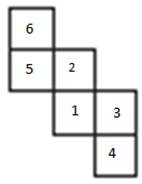i. Fold Square-6, 90° downwards

ii. Fold Square-5, 90° downwards

iii. Fold Square-4, 90° downwards

iv. Now, fold Square-3 90° downwards

v. Now, fold Square-1 90° downwards

Hence, by the above procedure we can form a cube with the given net.

Question 23.

(a) Name the polyhedron which has four vertices, four faces?

(b) Name the solid object which has no vertex?

(c) Name the polyhedron which has 12 edges?

(d) Name the solid object which has one surface?

(e) How is a cube different from cuboid?

(f) Which two shapes have the same number of edges, vertices, and faces?

(g) Name the polyhedron which has 5 vertices and 5 faces?

(a) A polyhedron with 4 vertices and 4 faces is tetrahedron.

(b) The only Solid object with no vertex is Sphere.

(c) The possible polyhedrons with 12 edges are a cube, cuboid, Hexagonal Pyramid.

(d) The Only solid object with 1 face is Sphere.

(e) Cube is a Regular Polyhedron (All faces are identical)

Whereas Cuboid is not a Regular Polyhedron (All faces are not identical)

(f) Cube and Cuboid have a same number of faces, edges, vertices.

That is 6 faces, 12 edges, 8 vertices.

(g) A Polyhedron with 5 vertices and 5 faces is square Pyramid.

Question 24.

Write the names of the objects given below.

(a)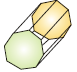(b)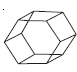(c)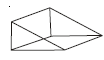(d)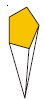(a) The given Object is Octagonal prism.

(b) The given Object is Hexagonal prism.

(c) The given Object is triangular prism.

(d) The given Object is Pentagonal Pyramid.

## MATHS VIDEOS